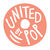Which Hogwarts House Do These Popular YA Characters Belong In?Created By United By Pop
On Feb 1, 2019

Lara Jean Song Covey (To All The Boy's I've Loved Before)

Calculating results

Peter Kavinsky (To All The Boy's I've Loved Before)

Calculating results

Starr Carter (The Hate U Give)

Calculating results

Willowdean Dickson (Dumplin')

Calculating results

Kaz Brekker (Six of Crows)

Calculating results

Nikolai Lantsov (King of Scars)

Calculating results

Nina Zenik (Six of Crows)

Calculating results

Inej Ghafa (Six of Crows)

Calculating results

Mare Barrow (Red Queen)

Calculating results

Cath Avery (Fangirl)

Calculating results

Levi Stewart (Fangirl)

Calculating results

Daniel/Day Altan Wing (Legend)

Calculating results

Monty (The Gentleman's Guide to Vice and Virtue)

Calculating results

Percy (The Gentleman's Guide to Vice and Virtue)

Calculating results

Katniss Everdeen (The Hunger Games)

Calculating results

Effie Trinket (The Hunger Games)

Calculating results

Simon Spier (Simon vs. the Homo Sapiens Agenda)

Calculating results

Leah Burke (Leah on the Offbeat)

Calculating results

Ruby Daly (The Darkest Minds)

Calculating results

Dimple Shah (When Dimple Met Rishi)

Calculating results

Magnus Bane (The Mortal Instruments)

Calculating results

Mona Vanderwaal (Pretty Little Liars)

Calculating results

Laia (An Ember in the Asher)

Calculating results